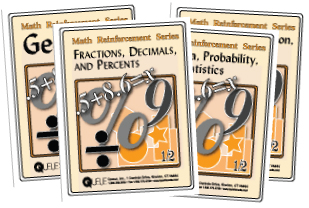Mathematics Reinforcement SeriesThe Mathematics Reinforcement Series offers your students approachable, targeted practice exercises designed to help them hone their skills in four areas:

• Fractions, Decimals, and Percents

Fractions: What are Fractions?, Recognizing Fractions, Identifying Fractions, Fractions of a Line, Finding Fractions by Shading, Finding a Fraction of a Number, and Finding Equivalent Fractions.

Decimals: Decimal Pieces, Equivalent Fractions and Decimals. Adding Decimals: Subtracting Decimals, Multiplying Decimals, and Dividing Decimals.

Percents: What is a Percent? and 5 Quizzes.

• Ratio and Proportion, Mass, and Time

Ratio and Proportion: Working out Ratios, Numbers to Ratios, Equivalent Ratios, Fraction Ratios, and Proportion.

Mass: Units of Mass, Estimating Mass, Converting Units, Adding Mass, Dividing Mass, and Making Mass Fit.

Time: Estimating Time, Units of Time, and Converting Time.

• Algebra, Probability, and Statistics

Algebra: Finding Rules, Number Patterns, Solving Problems with Equations, and Finding Variables.

Probability: Coin Toss, Throwing Dice, Measuring Chance, Predicting Spin.

Statistics: Surveys, Sampling, Sorting and Presenting Data, Picture Graphs, Pie Graph, Bar Chart, Column Graph, Histogram, Line Graph, Data Range, Data Mode, Data Median, Data Mean, Analyzing Results.

• Geometry

Length: Units of Length, Converting Units, Metric Conversions, Perimeters, Circumference and Diameter, and Discovering Pi. Area: Area of Rectangles, Area and Perimeter, Area of Triangles, Area of Circles, and Surface of a Cylinder.

Volume: Volume in Cubes, Volume.

#### Sample Pages

Choose from the following menu to download a PDF of a few sample pages from one of our Mathematics Reinforcement Series workbooks.

#### Product Codes for Mathematics Reinforcement Series

To better facilitate the ordering process, please use the following product codes when placing your order. Click here for information on interactive whiteboard versions.

 Subject Student Code Teacher Code Fractions, Decimals, and Percents QNZ 3100 QNZ 3101 Ratio and Proportion, Mass, and Time QNZ 3102 QNZ 3103 Algebra, Probability, and Statistics QNZ 3104 QNZ 3105 Geometry QNZ 3106 QNZ 3107

#### Prices and Ordering Information

Pricing

15-29 Student Workbooks: \$11.95 ea.

30+ Student Workbooks: \$10.95 ea.

FREE Teacher's Edition for every 30 copies of a single workbook title purchased